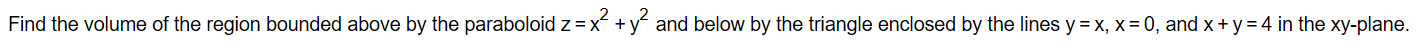# Find the volume of the region bounded above by the paraboloid z xad below by the triangle enclosed by the lines y x, x 0, and x + y 4 in the xy-plane.

Question
30 views

Question included in imagehelp_outlineImage TranscriptioncloseFind the volume of the region bounded above by the paraboloid z x ad below by the triangle enclosed by the lines y x, x 0, and x + y 4 in the xy-plane. fullscreen
check_circle

Step 1

Given,

The equation of the paraboloid z = x2 + y2

Lines are y = x, x = 0 and x + y = 4

And x-y plane

Step 2

Now, draw the graph is below:

Step 3

Formula us...

### Want to see the full answer?

See Solution

#### Want to see this answer and more?

Solutions are written by subject experts who are available 24/7. Questions are typically answered within 1 hour.*

See Solution
*Response times may vary by subject and question.
Tagged in

### Integration